# Calculating the Absolute Difference between Each List Value and the Minimum Value in the List

In this article, we are going to learn to calculate the Absolute difference between each list and the Minimum Value in the list.

To solve this problem, we will use ABS and MIN function.

• ABS function is used to return the absolute value of the number.
• MIN function will help to find out the minimum number within a range.

Let’s take an example and understand:-

We have number range in Column A. We want to find out the difference, from every number to minimum value.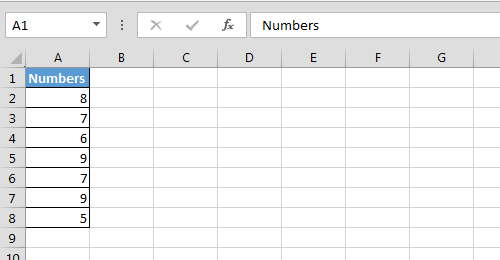• Enter the below formula in cell B2
• =ABS(MIN(\$A\$2:\$A\$8)-A2)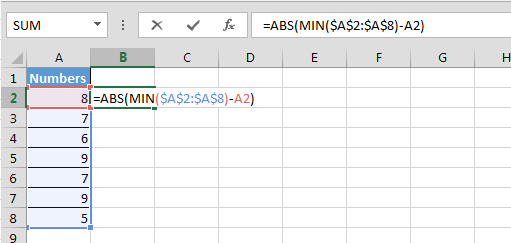• Copy the same formula in the range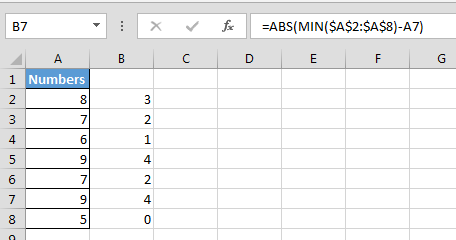In the above image, you can see the difference in the values from the minimum value.

Let’s take another example:-

Let’s say we have same number in vertical position then how will we carry out the same calculation?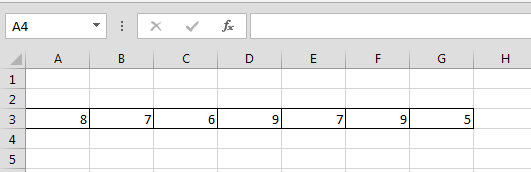• Enter the formula in cell A4
• =ABS(MIN(\$A\$3:\$G\$3)-A3)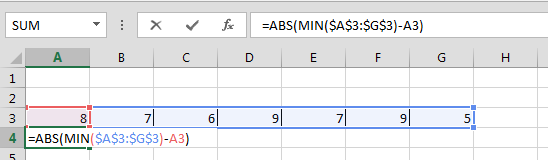• Copy same formula in the range B3:G3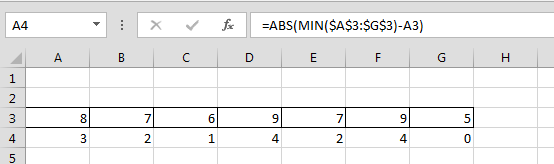Now you can see in the above image that we just have changed the cell reference and we are getting correct result.If you liked our blogs, share it with your friends on Facebook. And also you can follow us on Twitter and Facebook.

We would love to hear from you, do let us know how we can improve, complement or innovate our work and make it better for you. Write us at info@exceltip.com

Terms and Conditions of use

The applications/code on this site are distributed as is and without warranties or liability. In no event shall the owner of the copyrights, or the authors of the applications/code be liable for any loss of profit, any problems or any damage resulting from the use or evaluation of the applications/code.